Select Page

# Extended Operators in Relational Algebra

Extended operators are those operators which can derive from other operators i.e. (Unary or Binary operators).

## Types of Extended Operators

There are 3 types of extended operators in Relational Algebra

1. Intersection Operator
2. Divide Operator
3. Join Operator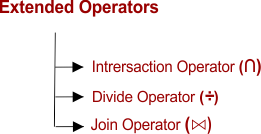### 1. Intersection Operator (∩)

Intersection operator is the reverse of Union operator. So, this Operator can derive from Union Operator. This operator can define as follows

Let A and B two relations.

Then

• A∩ B is the set of all tuples belonging to both A and B.
• In A∩ B, duplicates are automatically removed.
• Intersection operation is both commutative and associative.

Example

Consider the following two relations A and B

Relation A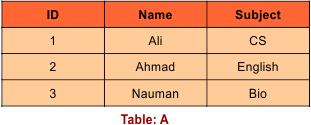Relation B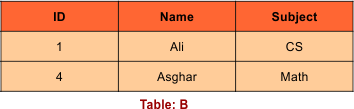Then, A ∩ B is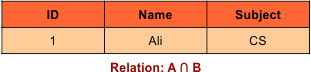### 2. Division Operator (÷):

Division operator (X÷Y) can apply if and only if:

• Attributes of Y is proper subset of Attributes of X

Proper Subset:

A={1,2,3} is a proper subset of B ={1,2,3,4} because the element 4 is not in the first set A.}

• After division operation, Remaining attributes in table will be = (All attributes of X – All Attributes of Y).
• After division operation, Relation will return those tuples from relation X which are associated to every tuple of Y.

Consider the following two tables STUDENT_SPORTS and SPORTS

Table: STUDENT_SPORTS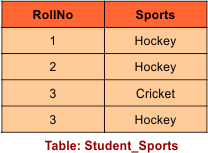Table: SPORTSAfter applying division operator (STUDENT_SPORTS÷ SPORTS)

• The division operation can apply as attributes in SPORTS table is a proper subset of attributes in STUDENT_SPORTS table.
• The Result After division operation, Remaining attributes in first table will be {ROLL_NO,SPORTS}-{SPORTS}=ROLL_NO
• The Roll_No column of Student_Sports table will have those values which are associated with all Y’s tuple {Hckey, Cricket}. ROLL_NO 1,2 are associated to Hockey only. ROLL_NO 3 is associated to all tuples of Y (Hockey and cricket). So, the resulting table will have the value “3” in Roll_No coloumn.

### 3. Join Operations:

A Join operation combines related tuples from different tables. Join operators can apply, if and only if a given join condition is satisfied. Join operator denoted by ⋈.

For join operation, there must be some common in two tables. As in below tables EMP_ID is common in both tables.

Join operation used when we need to access data from more than one tables.

In the following tables we need Emp_Name and EMP_Salary. SO it requires to join the EMPLOYEE and SALARY tables

Example: Suppose EMPLOYEE and SALARY Tables

Table: EMPLOYEE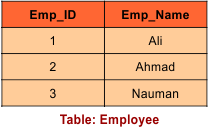Table: SALARYApply Join Operation (EMPLOYEE ⋈ SALARY)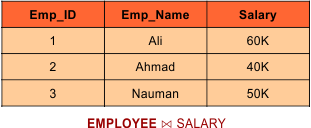## Types of JOIN Operator

1. Natural JOIN
2. OUTER JOIN
1. LEFT OUTER JOIN
2. RIGHT OUTER JOIN
3. FULL OUTER JOIN
3. Equi JOIN

In next lecture we will cover all above Types of Joins.

Help Other’s By Sharing…